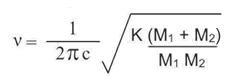For non-linear molecules, for example pentane, there are a number of vibrations given by (3N – 6). N is the number of atoms in the molecule. Pentane, C5 H12, (17 atoms) has 45 different vibrations!

Although there are a large number of vibrational modes here, the situation can be simplified by considering that each functional group can be considered independently. So a methyl group for example should have the same normal modes of vibration no matter where it is located in a molecule.

Water (H2 O) has three modes of vibration.

Carbon dioxide (CO2) is a linear molecule and produces just two peaks in the spectrum.

This is because the symmetric stretch does not have a dipole and the two bending vibrations (in plane and out of plane) are degenerate (i.e. of equal energy). The reason that only two peaks appear in the carbon dioxide spectrum is because the symmetrical stretching vibration does not have a change in dipole moment as it vibrates. The two bending vibrations are degenerate and vibrate at exactly the same frequency.

Other simple organic molecules produce a large number of peaks which could be considered in terms of those vibrations which correspond to CH3, CH2, benzene ring and the main functional groups e.g. alkene C=C, carbonyl C=O, alcoholic O-H, amine N-H etc. For example Vanillin.

The following video shows the spin rotation of the aspirin molecule.

Predicting the spectra of new molecules can be obtained by defining characteristic bonds, as shown by the regions of the IR spectrum here:

The approximate value for the frequency (ν cm-1) of absorption of a bond, can be calculated from the following (ν is referred to as wavenumber).Where c = velocity of light, k = force constant of a bond between atoms of masses M1 and M2.

For a very light atom such as hydrogen, the value for the term, (M1 +M2)/M1 M2 is approximately unity (~1.0) and has a relatively large value. The frequency for a C-H bond is approximately 3000 cm-1. Compare this value with that calculated for a bond between carbon and chlorine; here, (M1 +M2)/M1 M2 = 0.11; the frequency for a C-Cl bond is approximately 600-800 cm-1.

v (wavenumber) depends on the strength of the bond between the atoms M1 and M2 as given by K, the force constant.

### Types of Bonds

Values of the force constant for a number of bonds are given in the table below.

Bond      Force constant / dynes cm-1
C-C 4.5 X 105
C=C 9.6 X 105
C≡C 15.6 X 105
C=O 12.1 X 105
C≡N 17.73 X 105

This indicates that a C=C double bond is approximately twice as strong a s a C-C single bond and that a C≡C triple bond is approximately three times as strong as a C-C single bond, although there is no simple correlation between the force constant and the strength of a bond. Notice that the force constant is greater for C=O than C=C and greater for C≡N than C≡C. Clearly the bonds are stronger when the difference in electronegativity increases (and the subsequent dipole moment).

The strength (intensity) of an absorption in the infrared is dependent on the change of dipole moment occurring during the vibration. If there is no change in dipole moment then the radiation cannot interact with the vibration and there is no absorption.  Thus the C≡C stretching vibration does not appear in symmetrically substituted dialkylacetylenes.

2-methylbut-2-ene exhibits only a small dipole moment change and therefore absorbs very weakly. Carbonyl and similar highly polar groupings absorb strongly.

Absorption of single bonds to hydrogen e.g. C-H, N-H, O-H occur around 4000-2500 cm-1. For electronegative atoms i.e. oxygen and nitrogen, the peak will be broadened by intra or inter molecular hydrogen bonding. Absorption of triple bonds e.g. C≡C, C≡N (but also X=Y=Z) occur around 2500-2000 cm-1.

Absorption of double bonds e.g. C=O, C=C occur around 2000-1500 cm-1. These values fall to lower frequencies when bonds are conjugated (increasing single bond character). With carbonyl stretching frequencies, values are significantly affected by the inductive effect of an atom and by the mesomeric affect of groups bonded to the carbonyl carbon.

Absorptions occurring around 1500-400 cm-1 are due to other vibrations e.g. rotating, scissoring and some bending. This is referred to as the ‘fingerprint region’.

• #### Pentane vibrations

Simulation | Flash, Size 24.22 kb
• #### Water vibrations

Simulation | Flash, Size 20.82 kb
• #### Carbon dioxide vibrations

Simulation | Flash, Size 12.57 kb
• #### Vanillin vibrations

Simulation | Flash, Size 0.11 mb
• #### IR regions

Simulation | Flash, Size 81.05 kb

## Infrared (IR) spectroscopy

• 1
• 2
• 3
• 4Currently reading